Open in App
Not now

# Mahotas – Element Structure for Eroding Image

• Last Updated : 29 Jul, 2021

In this article, we will see how we can set the element structure for erode of the image in mahotas. Erosion (usually represented by ?) is one of two fundamental operations (the other being dilation) in morphological image processing from which all other morphological operations are based. It was originally defined for binary images, later being extended to grayscale images, and subsequently to complete lattices. In order to erode the image we use mahotas.morph.erode method.

In this tutorial, we will use “luispedro” image, below is the command to load it.

`mahotas.demos.load('luispedro')`

Below is the luispedro image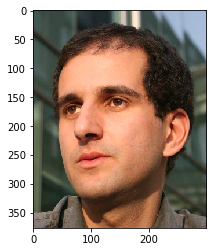Below is the default structure of the element for erosion, which a 1 cross

```np.array([
[0, 1, 0],
[1, 1, 1],
[0, 1, 0]],
bool)```

Below is the implementation

## Python3

 `# importing required libraries``import` `mahotas``import` `mahotas.demos``from` `pylab ``import` `gray, imshow, show``import` `numpy as np` `# loading image``luispedro ``=` `mahotas.demos.load(``'luispedro'``)` `# filtering image``luispedro ``=` `luispedro.``max``(``2``)` `# otsu method``T_otsu ``=` `mahotas.otsu(luispedro)`` `  `# image values should be greater than otsu value``img ``=` `luispedro > T_otsu` `print``(``"Image threshold using Otsu Method"``)` `# showing image``imshow(img)``show()` `# erode structure``es ``=` `np.array([``        ``[``1``, ``1``, ``1``],``        ``[``1``, ``1``, ``1``],``        ``[``1``, ``1``, ``1``]], ``bool``)`  `# eroding image using element structure``new_img ``=` `mahotas.morph.erode(img, es)` `# showing dilated image``print``(``"Eroded Image"``)``imshow(new_img)``show()`

Output :

`Image threshold using Otsu Method`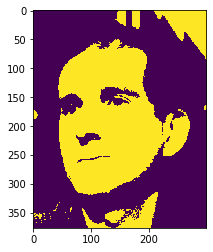`Eroded Image`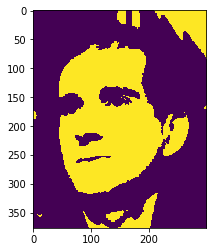Another example

## Python3

 `# importing required libraries``import` `mahotas``import` `numpy as np``import` `matplotlib.pyplot as plt``import` `os`` ` `# loading image``img ``=` `mahotas.imread(``'dog_image.png'``)``      ` `# setting filter to the image``img ``=` `img[:, :, ``0``]` `# otsu method``T_otsu ``=` `mahotas.otsu(img)`` `  `# image values should be greater than otsu value``img ``=` `img > T_otsu` `print``(``"Image threshold using Otsu Method"``)` `# showing image``imshow(img)``show()` `# erode structure``es ``=` `np.array([``        ``[``0``, ``0``, ``0``],``        ``[``0``, ``1``, ``0``],``        ``[``0``, ``0``, ``0``]], ``bool``)`  `# eroding image using element structure``new_img ``=` `mahotas.morph.erode(img, es)` `# showing dilated image``print``(``"Eroded Image"``)``imshow(new_img)``show()`

Output :

`Image threshold using Otsu Method `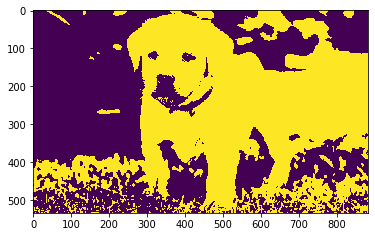`Eroded Image`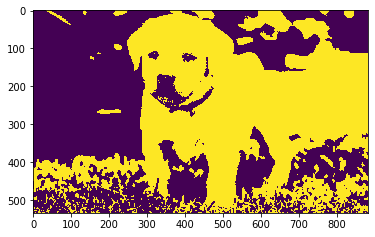My Personal Notes arrow_drop_up